Click to Chat

1800-1023-196

+91-120-4616500

CART 0

• 0

MY CART (5)

Use Coupon: CART20 and get 20% off on all online Study Material

ITEM
DETAILS
MRP
DISCOUNT
FINAL PRICE
Total Price: Rs.

There are no items in this cart.
Continue Shopping• Complete JEE Main/Advanced Course and Test Series
• OFFERED PRICE: Rs. 15,900
• View Details

```ICHO Past Year Question Papers 2012

1. This question is about sulfur chemistry
Sulfur forms many cyclic allotropes with different ring sizes. In the solid state, the most stable allotrope of sulfur is a form of S8. In the gas phase, all ring sizes from S3 to S12 have been detected.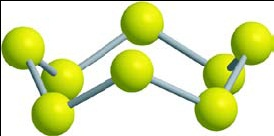In the gas phase, the different ring sizes are in equilibrium; the equation for the equilibrium between S7(g) and S8(g) is given below: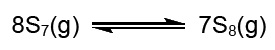(a) Given that the S–S bond strength in S7 is 260.0 kJ mol−1 and in S8 is 263.3 kJ mol−1, calculate the enthalpy change for the forward reaction.
When dissolved in an organic solvent, S6, S7 and S8 were all detected in equilibrium in the following proportions by mass:

ring size
S6
S7
S8

% by mass
0.32
0.76
98.92

(b) (i) Calculate the amount, in moles, of S7 and S8 at equilibrium when 1.00 g of sulfur is dissolved in 1.00 dm3 of solvent

(ii) ii) Give the expression for the equilibrium constant for the reaction between S7 and S8 as written above.

(iii) Calculate the value of this equilibrium constant.

In the solid phase, S8 crystallises in two well known forms: orthorhombic and monoclinic. The enthalpy changes of combustion of these two forms are as follows: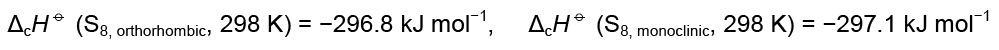(c) (i) Determine the enthalpy change at 298 K for the reaction(ii) Which is the more stable form at 298 K?
Sulfur also forms an 8-membered ring in a compound with nitrogen, S4N4, which forms goldcoloured crystals.
(d) In S4N4, nitrogen and sulfur atoms alternate in the ring. The nitrogen atoms form three bonds; two of the sulfur atoms form two bonds, two form four bonds. Draw the structure of S4N4 assuming there are no cross-links within the ring.
(e) An alternative form of the S4N4 structure is based on the same arrangement of atoms except there is a bond between both pairs of opposite sulfur atoms. Every sulfur atom has four bonds in this structure; nitrogen atoms again have three bonds. Draw this alternative structure of S4N4 (but do not attempt to draw the 3D structure).
(f) If S4N4 gas is passed over silver metal it yields a linear polymer, poly(sulfur nitride), that conducts electricity and is a superconductor at very low temperatures. The polymer contains just two types of bond: N−S and N=S. Each N atom has three bonds; each S has two or four. Draw a repeat unit of this polymer.

Human hair consists largely of proteins (polypeptides), such as keratin. Keratin is unusual in that it contains a very large proportion of the sulfur-containing amino acid cysteine.
The sulfur content of hair may be found by complete alkaline hydrolysis, followed by oxidation to convert all the sulfur to sulfate. This may be measured by precipitating it out as barium sulfate.A 1.00 g sample of hair is hydrolysed and the sulfur content converted to sulfate. Aqueous barium chloride is added until no more precipitation takes place, taking the total volume to 2.50 dm3. On filtration of the mixture at 20 °C, 260 mg of BaSO4 is recovered after drying.

(a) (i) Calculate the percentage by mass of sulfur in the sample of human hair to 3 significant figures. Assume that all the sulfur is converted to BaSO4 precipitate.
(ii) With such a small quantity of precipitate, account must be taken of its solubility inwater: 2.40 mg dm−3 at 20 °C. Calculate the percentage by mass of sulfur to 3 significant figures taking this solubility into account.

Cysteine is unique amongst amino acids in being able to form covalent cross-links betweenamino acids at two different points in a peptide chain. These covalent cross-links are called disulfide bonds, and two cysteine residues that have become linked in this way form a cystine residue. These disulfide bonds are responsible for the majority of the structural strength of hair.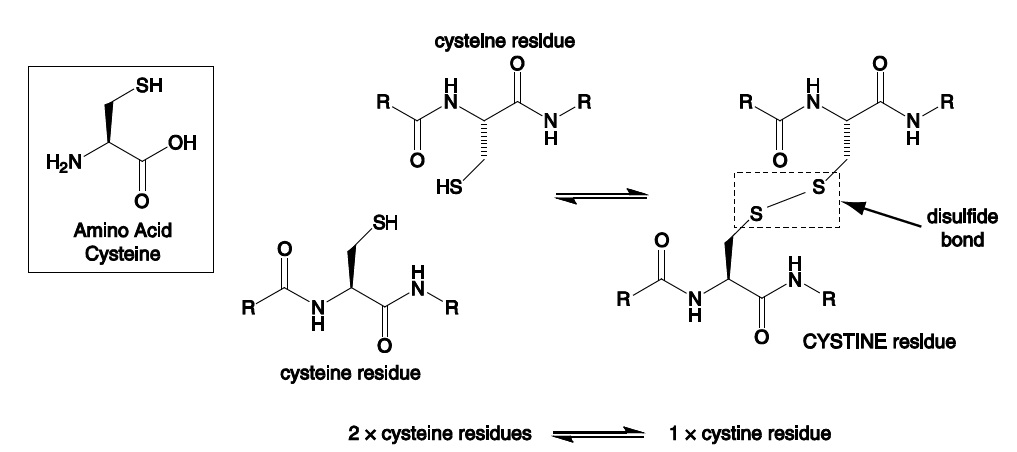(b) How would you classify this reaction of two cysteine residues to form a cystine residue?
(i) Dehydration (ii) Hydrolysis (iii) Oxidation (iv) Reduction
Both hair-perming lotions and hair removal cream use chemicals that break these disulfide bonds and so decrease hair strength. Both of these methods can use salts of thioglycolic acid in order to break the disulfide bonds.Thioglycolic acid has two acidic protons. The pKa values for the dissociation of these two protons are 3.67 and 10.31.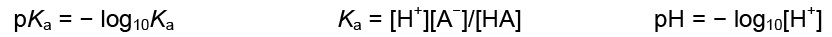(c) Draw the predominant form of thioglycolic acid that is present in aqueous solution at
(i) pH 0 (ii) pH 7 (iii) pH 14
(d) Calculate the percentage of mono-anion and percentage of di-anion present at pH 9.0. You may assume that these are the only two species present at this pH.
The chemical reaction carried out by the thioglycolic acid takes place in two steps:The following abbreviations are used:
RS-H:             thioglycolic acid (all forms, mono-anion, di-anion etc.)
Ker-S-S-Ker:    a cystine residue in keratin protein
Ker-S-H:         a cysteine residue in keratin protein.
(e) Write an equation for the overall chemical reaction.
As the initial disulfide bond cleavage is slow, it is often found that Step 1 is the rate determining step of the reaction. Usually the thioglycolic acid is present in a large excess and there is rapid diffusion into the hair. Under these reaction conditions the cleavage of the disulfide bonds follows second-order kinetics given by the equation below, but the concentration of thioglycolate is in a large excess and so remains essentially unchanged during the course of the reaction at concentration [RSH] 0. (The rate constant, k, is pH dependent and increases with increasing pH).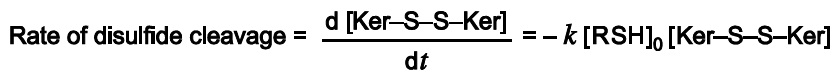(f) On the graph, which line best corresponds with how the concentration of Ker-S-S-Ker varies with time?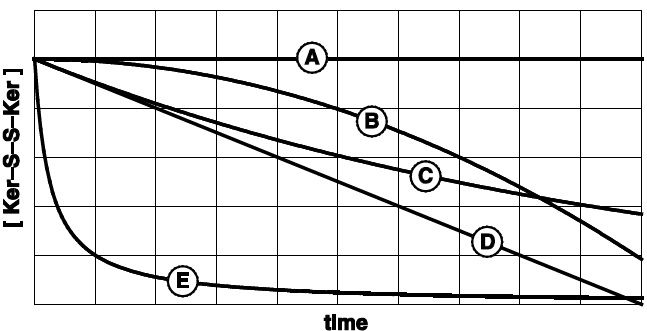In practice it is not possible to measure the number of Ker-S-S-Ker linkages directly. However, to a good approximation, the number of linkages is proportional to the tensile stress of a strand of hair, a property that can easily be measured mechanically. This allows the following equation to be derived relating the tensile stress, F, to the initial tensile stress, F0, and the time, t :Some strands of hair were treated with 0.16 mol dm–3 thioglycolate solution buffered at pH 9.0 for a period of one hour at 298 K.
(g) Use the graph below to calculate a value for the rate constant, k, at 298 K. Give the appropriate units.(h) Olympiad Perm Solution is a pH 9.0 solution containing ammonium thioglycolate and is available in 500 cm3 bottles. What is the mass of
ammonium thioglycolate in one bottle of Olympiad Perm Solution?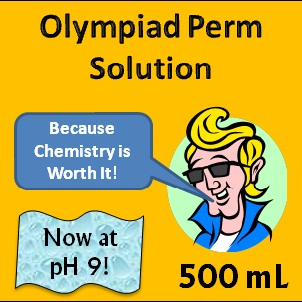3. This question is about chemistry general knowledge
(a) Put the following elements in the order in which they were discovered. Put the longestknown element first.
Ar          O          P          Pu          S
(b) Rank the following molecules in the order of their boiling point from 1 to 6. Rank the lowest-boiling-point "1" and the highest "6".
C2H5OC2H5        C2H5OH        HOCH2CH2OH        CH3CHO        C4H10        H2O
(c) Write the chemical formula that best describes the following.
(i) Fool’s gold          (ii) Epsom salts          (iii) Laughing gas
(d) What are the systematic names for the following chemicals?
(i) Acetone          (ii) Toluene          (iii) Sodium hypochlorite
(e) What is the colour change when zinc oxide is heated?
(f) Look at the chemical structures shown below. In the questions that follow, each compound should be assigned only once.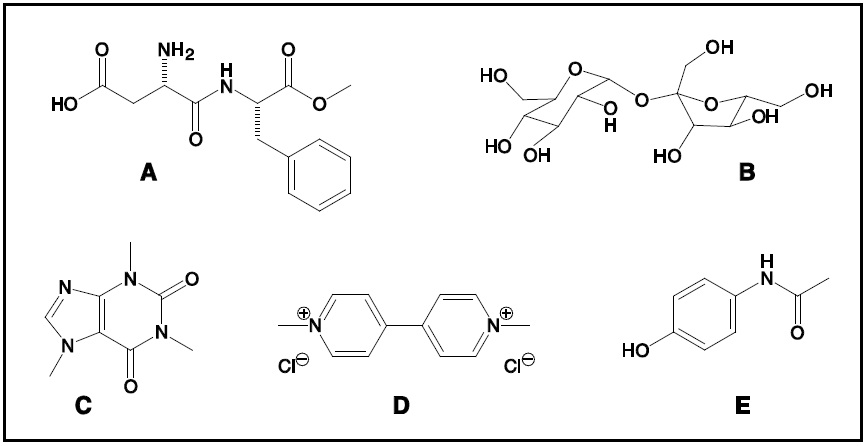(i) Which naturally occurring stimulant would you find in your tea?
(ii) Which might you take with a cup of tea if you have a headache?
(iii) Which compound might you add to your tea if you liked it sweet?
(iv) Which compound might you add to your tea if you liked it sweet but were on a low sugar diet?
(v) Which compound would you never add to a cup of tea?
4. This question is about the synthesis of top-selling drug Lipitor?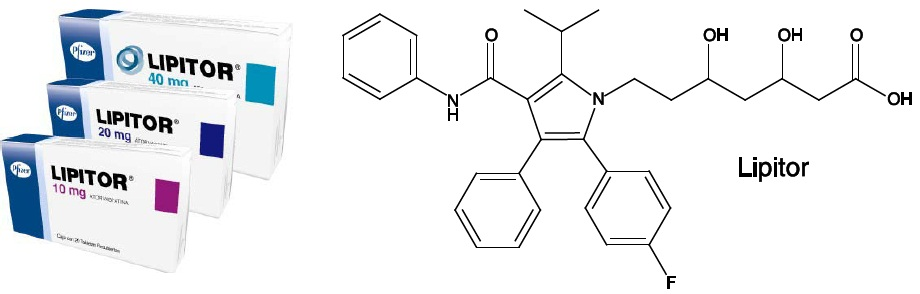With annual sales worth billions of pounds, for many years Pfizer's cholesterol-lowering drug Lipitor has been the best-selling pharmaceutical in the world. The first steps of a multi-stagesynthesis of Lipitor are shown below.
Given in the boxes are the most characteristic IR stretching frequencies of the intermediatesin the synthesis. No stretches due to any C–C or C–H bonds are included; stretchingfrequencies due to single bonds other than bonds to hydrogen do not show up in the range listed. You are not expected to know these stretching frequencies, but through careful reasoning, you should be able to use them to help work out the structures of the unknowns.

Note that not all the by-products are shown in the reaction schemes.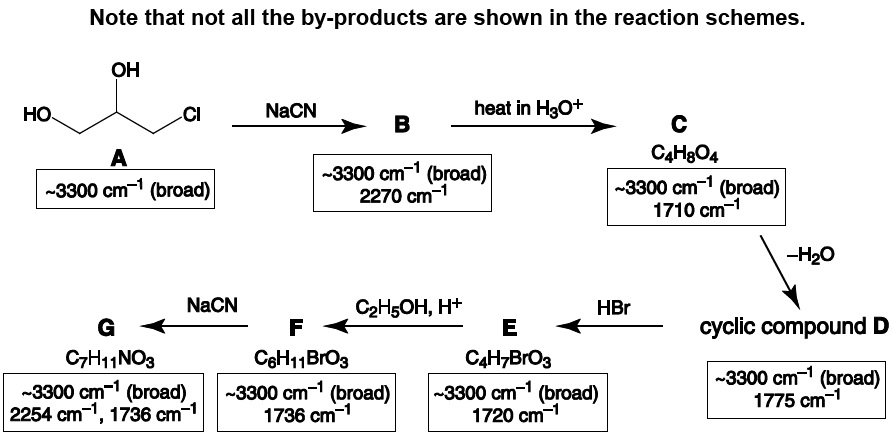(a) Give the structures for the compounds B to G and, over the course of the whole question, complete the table of IR absorptions found in compounds A to M.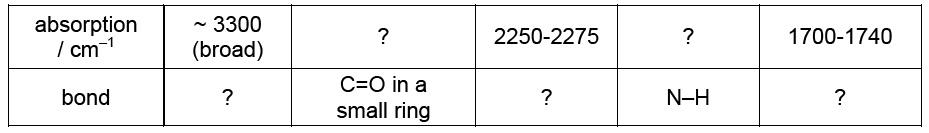Ester H is deprotonated by strong bases to give the reactive carbon nucleophile, anion I. The R group in the structure is an alkyl chain which remains unchanged throughout the entire synthesis.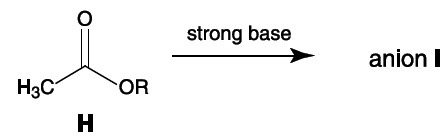(b) Draw the structure for the anion I.
The synthesis continues as shown below: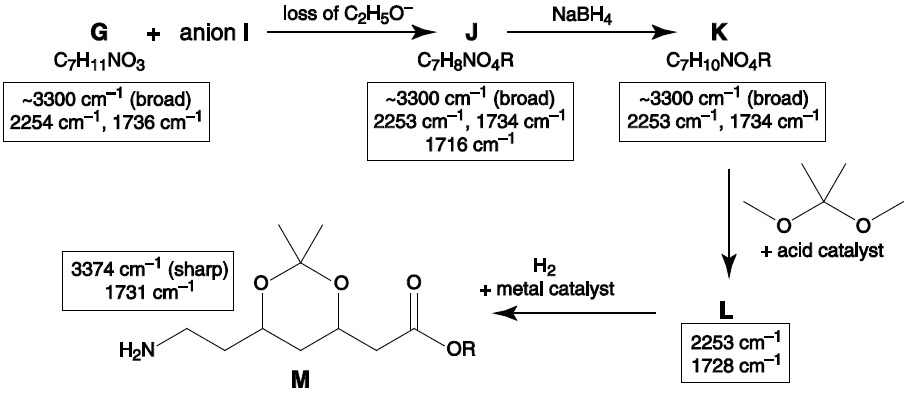(c) Give the structures for the compounds J, K and L.
In a separate branch of the synthesis, N reacts with phenylamine to give compound O. This may be deprotonated in base to give another carbon nucleophile, anion P. Anion P reacts with benzaldehyde to give Q ; Q then dehydrates to give compound S. S reacts with fluorobenzaldehyde in the presence of a catalyst to give compound T.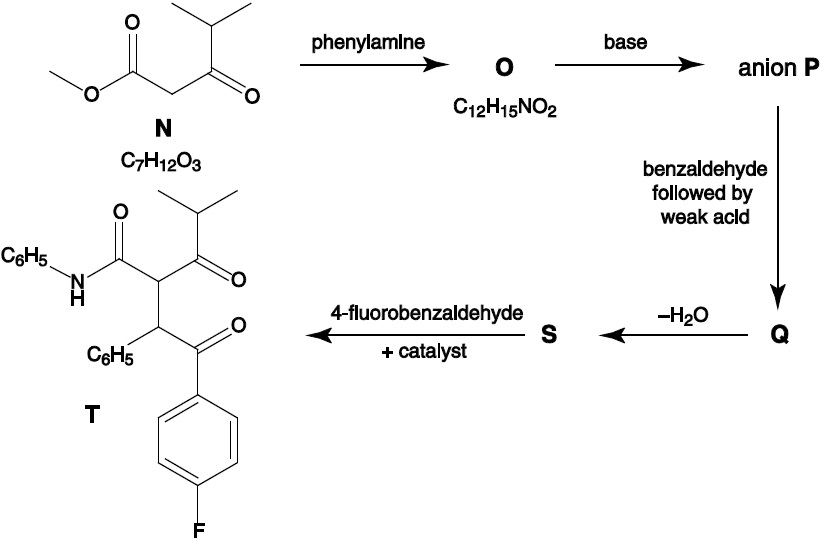(d) Give the structures of phenylamine and 4-fluorobenzaldehyde.
(e) Suggest structures for O, anion P, and compounds Q and S.
In the final stage of the synthesis, T and M are brought together to give compound U which on hydrolysis in aqueous acid gives the target, the drug Lipitor.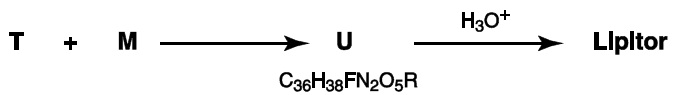(f) Suggest a structure for compound U.
5. This question is about the world's smallest powered car
In November of 2011, the premier scientific journal Nature published an article titled "Electrically driven directional motion of a four-wheeled molecule on a metal surface". Essentially, this paper reported the synthesis and observations of the world's smallest powered car – the "Nano-car".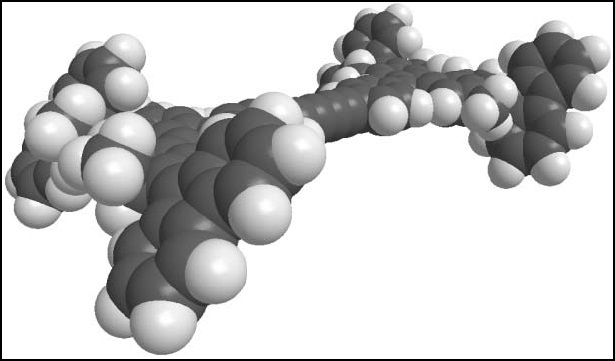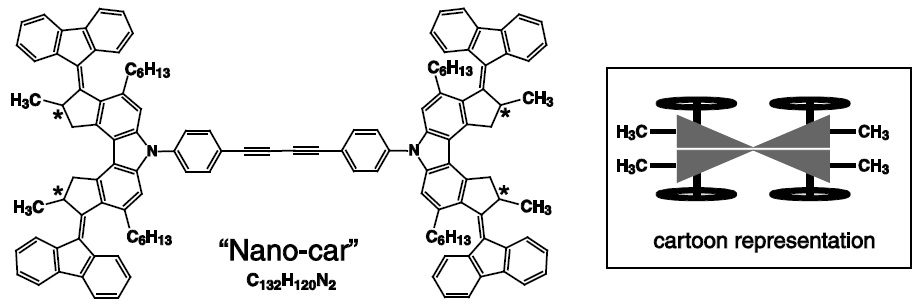On completion of the synthesis, the sample was analysed using NMR and mass spectrometry. Not surprisingly, the group did not submit their precious material for combustion analysis!
(a) (i) Give an equation for the complete combustion of nano-car.
(ii) Calculate the percentage by mass of carbon, hydrogen and nitrogen that would be predicted were the analysis to be performed. Give your answers to two decimal places.
Crucial in the design of the molecule are the hexyl and methyl groups shown explicitly in the structure above. It is because of these that the aromatic "wheels" of the car are actually arranged at an angle, straining the double bond that attaches them to the rest of the structure. This double bond can break, rotate and reform when sufficient electronic energy is provided using the tip of a scanning tunnelling microscope.
The carbon atoms to which the methyl groups are attached are chiral centres – each has four different groups attached to it. These are marked with an asterisk in the structure above.
The car is made up of four rotor units. A separate rotor unit exists as optical isomers – non-superimposable mirror images called enantiomers. Chemists distinguish between them with the labels "R" and "S" as shown on the right. The "wheels" on each enantiomer turn in opposite directions as shown (as viewed as if standing next to the car facing the wheel).In the synthesis of the car, a number of different stereoisomers were formed. These are shown in cartoon form below, in the arrangements the chemists detected on a surface of copper atoms (as viewed from above).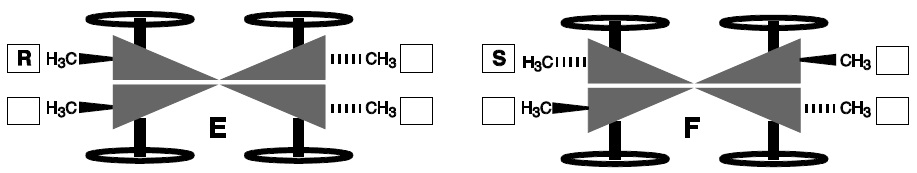(b) In your answer booklet, assign the label "R" or "S" to each rotor unit in the cars A-F in the boxes provided. The top left rotor in each car has already been assigned.
(c) Which of the cars A-F are optical isomers?
(d) Before being deposited on the copper surface, rotation is possible about the triple bonds linking the two halves of the car. Taking this into account, which of the cars A-F are the same molecule when free from the surface?
(e) By considering the directions of rotation for the four rotor units in each car, and assuming that all four rotors are active simultaneously, complete the table in the answer booklet, indicating whether each car will :

spin round on the copper surface clockwise

spin round on the copper surface anti-clockwise

remain stationary on the copper surface

move forwards over the copper surface.

```### Course Features

• 728 Video Lectures
• Revision Notes
• Previous Year Papers
• Mind Map
• Study Planner
• NCERT Solutions
• Discussion Forum
• Test paper with Video Solution